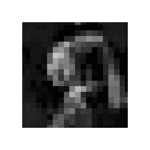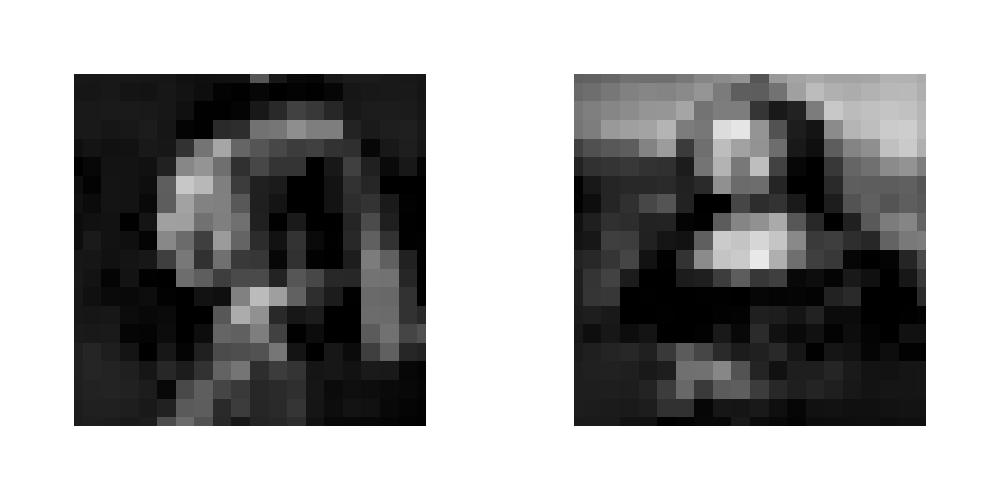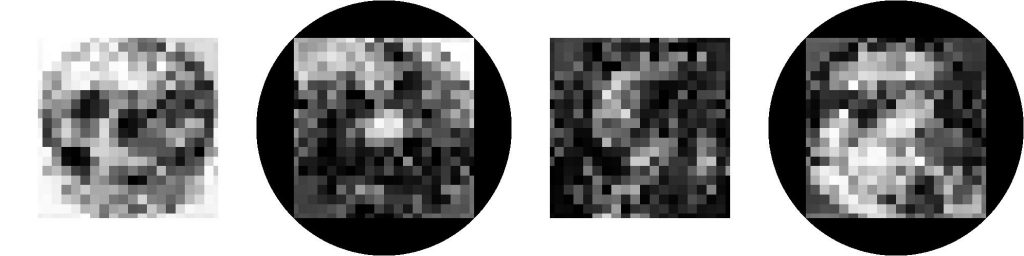Categories

# Polarization Images

Have you ever played with polarized films? If they’re aligned, they look transparent. If they-re misaligned by 90 degrees, they become opaque. Let’s use this to make pictures!

The intensity of transmission is governed by Malus’s Law, which says that the intensity is $cos(\Delta\theta)^2$, where $\Delta\theta=\theta_1-\theta_2$ is the misalignment of the two films. Now how can we use this to make images?

Forone image, with brightness $I_1(x,y)$, it’s easy. Have one film with a constant orientation, $\theta_2=0$, then $\theta_1=\arccos(\sqrt{I_1})$. But that’s boring.

What about multiple layers? Let’s say that we make the first image by overlapping the films normally, and the second by rotating film 2 by 180 degrees. The brightness of the new images, $J$, is

\begin{aligned} J_1(x,y) &= \cos\left[ \theta_1(x,y)- \theta_2(x,y) \right]^2 \\ J_2(x,y) &= \cos\left[ \theta_1(x,y)-\left( \theta_2(-x,-y)-\pi \right) \right]^2 \\ &= \cos\left[ \theta_1(x,y)-\theta_2(-x,-y)\right]^2 \end{aligned}

The rotation means that points are paired in an important way: the brightness at points (x,y) and (-x,-y) depend on the film orientations at both points. So the problem can be independently solved for every pair of rotation-linked points.

Unfortunately, it isn’t easy to get simple analytic solutions. So let’s do some optimization. Define an error function

$\epsilon = \sum_{x,y}\left[ (I_1-J_1)^2+(I_2-J_2)^2 \right]$,

and throw random search at it. That works really well for finding a rough approximation, then gradient descent can fine-tune it.

Alternatively, you can find the rough approximation by noticing that you can divide the problem into sections. Say A is the left side, and B is the right side. Since the images are generated as differences, you can set one region to zero and only optimize the rest, like $\theta_2(B)=0$. Then you can create a 3D grid of each possible $\Theta =[\theta_1(A), \theta_1(B), \theta_2(A)]$ and find the resulting values of $J_A(\Theta),J_B(\Theta)$. With these, you can pick a good starting point for gradient descent for each rotation-pair.

Check out the result with the Mona Lisa and Girl with a Pearl Earring. First, here’s a a rough version that shows the individual squares. They are mounted on circular backings of constant orientation, and are placed atop a background field that’s misaligned by 17 degrees, just to show their outlines.Rotating through 360 degrees, pausing at 0 and 180 degrees.Here are the stills at 0 and 180 degrees.

It works! What about higher resolution? The following image shows the original image, recreation, and ten times the absolute difference between the two. You can see that there are some inexact regions, but it does a good job generally!

Using two images is good, but why not four? Apply the same general thought process, and it still works! The image quality is substantially worse, but it does seem to work.Can you tell what these are? Probably not easily.